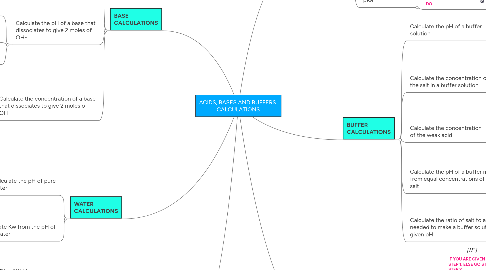ACIDS, BASES AND BUFFERS CALCULATIONS

For A level Chemistry (OCR, AQA, CIE and Edexcel).

Get Started. It's FreeACIDS, BASES AND BUFFERS CALCULATIONS1.1.1. STEP 1

1.1.2. STEP 2

1.2.1. STEP 1

1.2.2. STEP 2

1.3.1. STEP 1

1.3.2. STEP 2

1.4.1. STEP 1

1.4.2. STEP 2

1.5.1. STEP 1

1.5.2. STEP 2

2. STRONG ACID CALCULATIONS

2.1.1. STEP 1

2.1.2. STEP 2

2.2.1. STEP 1

2.2.2. STEP 2

2.3. Calculate the pH of a monoprotic strong acid

2.3.1. THIS IS ALL YOU NEED TO DO

2.4. Calculate the concentration of s monoprotic Strong acid

2.4.1. THIS IS ALL YOU NEED TO DO

3. WEAK ACID CALCULATIONS

3.1. Calculate Ka

3.1.1. IF YOU ARE GIVEN pH THIS IS STEP 1, ELSE GO STRAIGHT TO STEP 2

3.1.2. STEP 2

3.2.1. STEP 1

3.2.2. STEP 2

3.3.1. STEP 1

3.3.2. STEP 2

4.1.1. STEP 1

4.1.2. STEP 2

4.2.1. STEP 1

4.2.2. STEP 2

4.3.1. STEP 1

4.3.2. STEP 2

4.3.3. STEP 3

4.4.1. STEP 1

4.4.2. STEP 2

4.4.3. STEP 3

5.1.1. STEP 1

5.1.2. STEP 2

5.2.1. STEP 1

5.2.2. STEP 2

6. PKa CALCULATIONS

6.1. Calculate pKa

6.1.1. IF YOU ARE GIVEN Ka

6.1.2. IF YOU DON'T HAVE Ka

6.1.2.1. STEP 1

6.1.2.2. STEP 2

6.2. Calculate Ka from pKa

6.2.1. THIS IS ALL YOU NEED TO DO